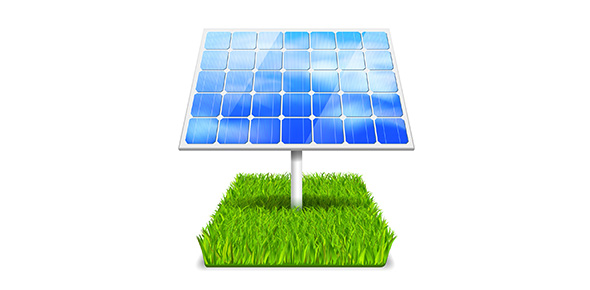# Miss Ashurst - Core Physics - Energy Transfer

10 Questions | Total Attempts: 62SettingsCreate your own Quiz• 1.
As a space shuttle moves towards Earth, it loses.......
• A.

Gravitational potential energy

• B.

Kinetic energy

• C.

Light energy

• D.

Thermal energy

• E.

Sound energy

• 2.
When a car moves faster it gains......
• A.

Gravitational potential energy

• B.

Kinetic energy

• C.

Light energy

• D.

Thermal energy

• E.

Sound energy

• 3.
The input energy into a mobile phone is.........energy.
• A.

Electrical

• B.

Thermal

• C.

Light

• D.

Sound

• E.

Kinetic

• 4.
The useful energy output from the speaker is ..........energy.
• A.

Electrical

• B.

Light

• C.

Thermal

• D.

Sound

• E.

Kinetic

• 5.
The useful energy output from the screen is .......... energy.
• A.

Electrical

• B.

Light

• C.

Thermal

• D.

Sound

• E.

Kinetic

• 6.
The wasted energy output from the mobile phone is........energy.
• A.

Electrical

• B.

Light

• C.

Thermal

• D.

Sound

• E.

Kinetic

• 7.
In an eco-friendly house solar cells transfer........
• A.

Chemical energy to thermal energy

• B.

Electrical energy to thermal energy

• C.

Light energy to electrical energy

• D.

Kinetic energy to electrical energy

• E.

Light energy to sound energy

• 8.
In an eco-friendly house a wind turbine transfers........
• A.

Chemical energy to thermal energy

• B.

Electrical energy to thermal energy

• C.

Light energy to electrical energy

• D.

Kinetic energy to electrical energy

• E.

Light energy to sound energy

• 9.
In an eco-friendly house biofuel used in the cooker transfers........
• A.

Chemical energy to thermal energy

• B.

Electrical energy to thermal energy

• C.

Light energy to electrical energy

• D.

Kinetic energy to electrical energy

• E.

Light energy to sound energy

• 10.
As a tidal barrage used to generate electricity moves water moves from a lower level to a higher level it gains mainly.......
• A.

Electrical energy

• B.

Gravitational potential energy

• C.

Sound energy

• D.

Thermal energy

• E.

Light energy

Related TopicsBack to top
×

Wait!
Here's an interesting quiz for you.